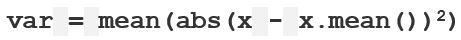# numpy.nanvar() in Python

• Last Updated : 03 Dec, 2018

`numpy.nanvar(arr, axis = None)` : Compute the variance of the given data (array elements) along the specified axis(if any), while ignoring NaN values.Example :

x = 1 1 1 1 1
Standard Deviation = 0 . Variance = 0

y = 9, 2, 5, 4, 12, 7, 8, 11, 9, 3, 7, 4, 12, 5, 4, 10, 9, 6, 9, 4

Step 1 : Mean of distribution 4 = 7
Step 2 : Summation of (x – x.mean())**2 = 178
Step 3 : Finding Mean = 178 /20 = 8.9
This Result is Variance.

Parameters :
arr : [array_like] input array.
axis : [int or tuples of int]axis along which we want to calculate the variance. Otherwise, it will consider `arr `to be flattened (works on all the axis). axis = 0 means variance along the column and axis = 1 means variance along the row.
out : [ndarray, optional]Different array in which we want to place the result. The array must have the same dimensions as expected output.
dtype : [data-type, optional]Type we desire while computing variance.

Results : Variance of the array (a scalar value if axis is none) or array with variance values along specified axis; while ignoring NaN values.

Code #1:

 `# Python Program illustrating  ` `# numpy.nanvar() method  ` `import` `numpy as np  ` `     `  `# 1D array  ` `arr ``=` `[``20``, ``2``, np.nan, ``1``, ``34``]  ` ` `  `print``(``"arr : "``, arr)  ` `print``(``"\nnanvar of arr : "``, np.nanvar(arr))  ` ` `  `print``(``"var of arr : "``, np.var(arr))  ` ` `  `print``(``"\nnanvar of arr : "``, np.nanvar(arr, dtype ``=` `np.float32))  ` `print``(``"var of arr : "``, np.var(arr, dtype ``=` `np.float32))  ` ` `

Output :

```arr :  [20, 2, nan, 1, 34]

nanvar of arr :  187.1875
var of arr :  nan

nanvar of arr :  187.1875
var of arr :  nan```

Code #2:

 `# Python Program illustrating  ` `# numpy.nanvar() method  ` `import` `numpy as np  ` `     `  ` `  `# 2D array  ` `arr ``=` `[[``2``, ``2``, ``2``, ``2``, ``2``],  ` `    ``[``15``, ``6``, np.nan, ``8``, ``2``],  ` `    ``[``23``, ``2``, ``54``, ``1``, ``2``, ],  ` `    ``[np.nan, ``44``, ``34``, ``7``, ``2``]]  ` ` `  `     `  `# nanvar of the flattened array  ` `print``(``"\nnanvar of arr, axis = None : "``, np.nanvar(arr))  ` ` `  `print``(``"\nvar of arr, axis = None : "``, np.var(arr))  ` ` `  `     `  `# nanvar along the axis = 0  ` `print``(``"\nnanvar of arr, axis = 0 : \n"``, np.nanvar(arr, axis ``=` `0``))  ` ` `  `print``(``"\nvar of arr, axis = 0 : "``, np.var(arr, axis ``=` `0``))  ` ` `  `# nanvar along the axis = 1  ` `print``(``"\nnanvar of arr, axis = 1 : "``, np.nanvar(arr, axis ``=` `1``))  `

Output :

```nanvar of arr, axis = None :  249.88888888888889

var of arr, axis = None :  nan

nanvar of arr, axis = 0 :
[ 74.88888889 312.75       458.66666667   9.25         0.        ]

var of arr, axis = 0 :  [   nan 312.75    nan   9.25   0.  ]

nanvar of arr, axis = 1 :  [  0.      22.1875 421.84   313.1875]```

My Personal Notes arrow_drop_up
Recommended Articles
Page :